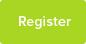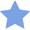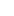# Tutor Hunt Questions

For any positive real number, show that:

1<a/Sqrt(a^2+b^2)+b/Sqrt(b^2+c^2)+c/Sqrt(c^2+a^2)

3/Sqrt(2)>a/Sqrt(a^2+b^2)+b/Sqrt(b^2+c^2)+c/Sqrt(c^2+a^2)
3 years ago

Question is vague .....a, b, c are they all positive real numbers?
19/02/2016 13:28:08 | comment by Alan

Please enter your response to the question below. The student will get a notification as soon your response has been approved by our moderation team.

####Accepted Answer

>>Right Hand Side:
The three term
F(a) = a/sqrt(a^2+b^2)
F(b) = b/sqrt(b^2+c^2)
F(c) = c/sqrt(c^2+a^2)
No matter how we order a, b and c, we can always have two of the terms F(a), F(b) and F(c) above or below 1/sqrt(2) at the same time.

Let`s assume the two terms are F(a) and F(b):
[F(a)-1/sqrt(2)]*[F(b)-1/sqrt(2)]>=0.

Therefore:
F(a)/sqrt(2)+F(b)/sqrt(2)
<=F(a)F(b)/2+1/2
<=ab/sqrt[(a^2+b^2)*(b^2+c^2)]+1/2
<=ab/(ab+bc)+1/2=a/(a+c)+1/2
=3/2-c/(a+c)<=3/2-c/sqrt(a^2+c^2)sqrt(2)

Therefore:
[F(a)+F(b)+F(c)]/sqrt(2)<=3/2

>>Left Hand Side:
F(a)+F(b)+F(c)
=1/sqrt(1+b^2/a^2)+1/sqrt(1+c^2/b^2)+1/sqrt(1+a^2/c^2).
Let x=b^2/a^2, y=c^2/b^2 and z=a^2/c^2

Using xyz=1, we have:
F(a)+F(b)+F(c)
=1/sqrt(1+x)+1/sqrt(1+y)+1/sqrt(1+z)
>=1/(1+x)+1/(1+y)+1/(1+z)
=(3+2x+2y+2z+xy+xz+yz)/[(1+x)(1+y)(1+z)]
>=(1+xyz+x+y+z+xy+xz+yz)/[(1+x)(1+y)(1+z)]
=1

Answered by Jett | 3 years ago
Excellent!
23/02/2016 18:18:32 | comment by Alin
As Ojuade wrote its straightforward, choose a=b=c=1/2
you will get the right hand side equal to 1.5, and 1<1.5.
3/sqrt(2)=2.1232 >1.5.

hope this help.
Answered by Rewayda | 3 years ago
1,2,3,4,5,... are natural numbers. The question asks you to prove for any positive REAL number.
07/09/2016 16:40:05 | comment by Ahmed
This is pretty straightforward.
Positive real numbers are 1,2,3,4,5....
Now, let a=1, b=2, c=3 and substituting these into the equation

a/Sqrt(a^2+b^2)+b/Sqrt(b^2+c^2)+c/Sqrt(c^2+a^2)

= 1/√(5) + 2/√(13) + 3/√(10) = 1.95

This clearly shows that:

1<1.95
and
3/√(2) = 2.12 which is > 1.95
You don`t even understand the problem, do you?
21/02/2016 22:10:56 | comment by Alin
Suppose that a>0, b> and c>0.

Now for each term:

a/Sqrt(a^2+b^2) has a solution between (0,1),
b/Sqrt(b^2+c^2) has a solution between (0,1),
c/Sqrt(c^2+a^2) has a solution between (0,1).

Taking the limit as a, b and c to reach infinity, we obtain:

a/Sqrt(a^2+b^2) approaches cos(pi/4)=1/sqrt(2),
b/Sqrt(b^2+c^2) approaches cos(pi/4)=1/sqrt(2),
c/Sqrt(c^2+a^2) approaches cos(pi/4)=1/sqrt(2).

Summing up all three terms above, we get an equation which satisfies:

a/Sqrt(a^2+b^2)+b/Sqrt(b^2+c^2)+c/Sqrt(c^2+a^2)<3/sqrt(2), where:

1/sqrt(2)+1/sqrt(2)+1/sqrt(2)=3/sqrt(2).

Hope this helps :)

Answered by Yacine | 3 years ago
This does not even prove half of the problem. And you way of thinking is wrong.
21/02/2016 22:11:52 | comment by Alin
Here was my alternative way: Let f(a,b,c)=a/Sqrt(a^2+b^2)+b/Sqrt(b^2+c^2)+c/Sqrt(c^2+a^2), Case 1: Suppose a=b, we get: f(a,c)=1/sqrt(2)+(a+c)/sqrt(a^2+c^2) If a is different to c, then: f(a,c) has a solution between 1+1/sqrt(2) and 3/sqrt(2). If a=c, then: f(a)=3/sqrt(2). So this is surely the upper bound. Case 2: Suppose a, b and c are different. If a was very small, b very large and c very large, then we expect f(a,b,c) to approach 1, i.e. the lower bound. A mixture of values would give us a maximum of 3/sqrt(2). Hence, 1<f(a,b,c)<3/sqrt(2).
22/02/2016 15:13:51 | comment by Yacine